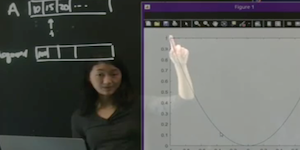# Data Science for Biology

## Introduction to MATLAB and Computer Basics

### Lecture 1### [ Part 1 ] [ 2 ] [ 3 ] [ 4 ]

INTRODUCTION TO MATLAB: The following lectures introduce the MATLAB software package and is basic functionality.

MATLAB COMMANDS

`SIZE`

### Lecture 2### [ Part 1 ] [ 2 ] [ 3 ] [ 4 ]

LOOPS AND LOGIC: The structure of logic protocols and iterative loops are constructed in MATLAB.

MATLAB COMMANDS

`FOR IF`

### Lecture 3### [ Part 1 ] [ 2 ] [ 3 ]

IMPORTING AND EXPORTING DATA: We demonstrate how to load and export data in MATLAB.

MATLAB COMMANDS

`LOAD SAVE`

MATLAB CODE